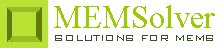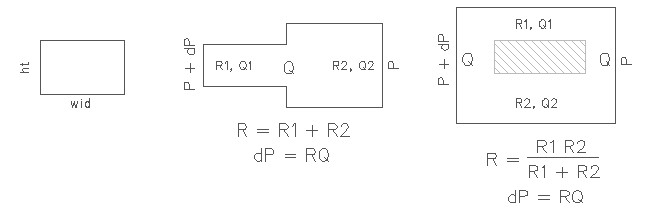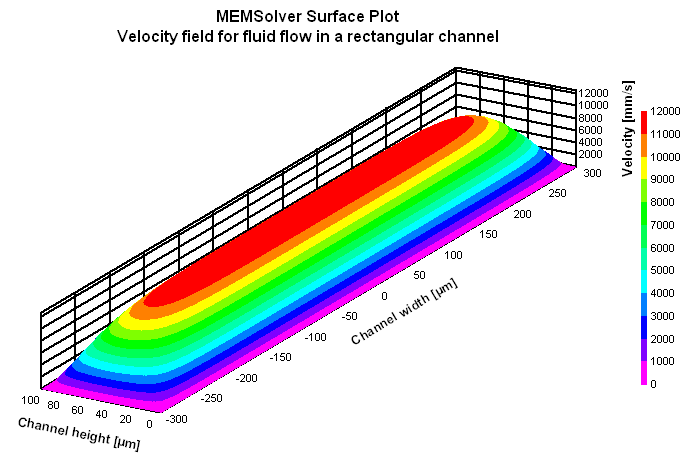RETCHN,len,wid,ht,dP,vis,sel
Design of a microfluidic channel with rectangular cross section

len        length of the channel in µm
wid        width of the channel in µm
ht          height or depth of the channel in µm
dP         applied pressure difference over the channel length in kPa
vis        dynamic viscosity in mPa-s
sel        number denoting the selected result.
Use 1 for hydraulic flow resistance, 2 for flow rate and 3 for average flow velocityNotes

Pressure driven, steady state flow of an incompressible fluid through a straight microchannel is typically laminar in nature, where the viscous effects dominate and inertial effects can be neglected as characterized by very small Reynolds's number. Viscous effects leads to internal friction in the fluid and creates resistance to the flow of the fluid. Just like electrical resistance leads to Joule heating, hydraulic resistance leads to dissipation of kinetic energy into heat.

Understanding hydraulic resistance is vital to the design of a microchannel. Mathematically it is represented by the Hagen-Poiseuille law that relates constant pressure drop dP resulting in a constant fluid flow Q

dP = Rhyd Q

Hagen-Poiseuille law is analogous to the Ohm's law dV = RI that relates the current I through a wire of resistance R and an electrical potential drop of dV. Similar to electrical resistors connected in series, two channels with different hydraulic resistance can be modeled by summing the two hydraulic resistance together as shown in the picture above. On similar lines, two hydraulic resistors connected in parallel follows the law of additivity of inverse of their hydraulic resistances as shown in the figure.

Use this design form to calculate the hydraulic resistance of a rectangular microfluidic channel with constant cross sectional area. If the channel has varying cross sectional area, the hydraulic resistance for individual channels with constant cross section can be found using this design form and then added together using the additivity law explained above. The flow rate can then be estimated using the Hagen-Poiseuille law. The average flow velocity is approximately one half of the maximum velocity which is at the center of the channel. To find the time the fluid takes to travel the full length of the channel, divide the length of the channel with the flow velocity.The plot shows the velocity field for the Poiseuille flow in a rectangular channel. The plot in blue color shows the velocity field along the center line parallel to the width of the channel. This plot is Vx(y,ht/2) shown as Vxy in the plot and picture above. The plot in red color shows the velocity field along the center line parallel to the height of the channel. This plot is Vx(0,z) shown as Vxz in the plot and picture above. The maximum velocity can be found out from the velocity field plot which happens at the center of the channel.Assumptions

-The Reynold's number is small Re << 1 and inertial effects can be neglected.
-The channels are long, rigid and narrow.
-The channel width is much larger than its height.
-No-slip boundary condition is assumed.
-The fluid has constant viscosity.
-Perfect hydrostatic pressure balance is assumed in the plane of the channel.